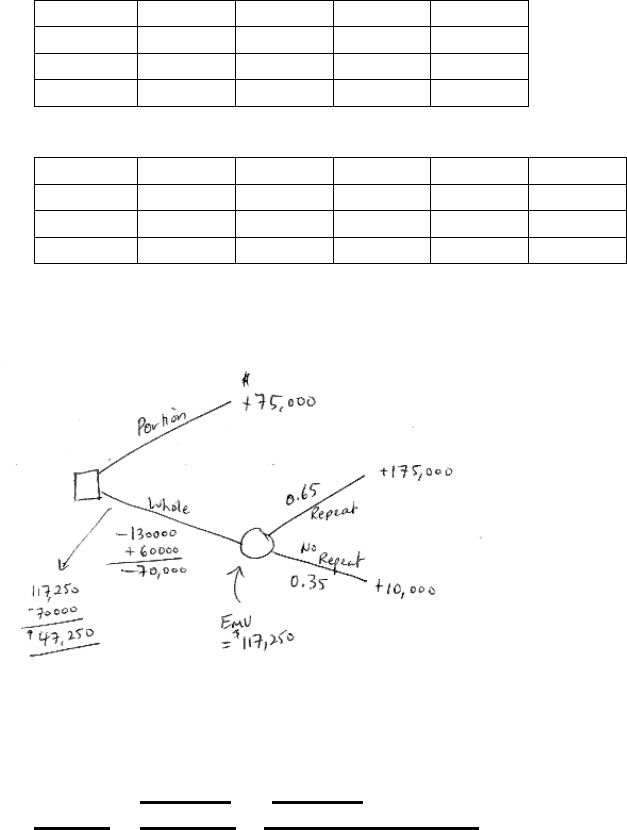Study Guides (380,000)
CA (150,000)
SFU (4,000)
BUS (700)
BUS 336 (10)
Final

# BUS 336 Study Guide - Final Guide: Shadow Price, Electronic Component, Expected Value Of Perfect Information

Department
Course Code
BUS 336
Professor
Michael Favere- Marchesi
Study Guide
Final

This preview shows pages 1-2. to view the full 7 pages of the document.BUS 336 Review
1 of 7
1. a) you should have no problem setting this up ….. if you do come to one of the open labs!
b) EVDELTA = \$102,000 is the highest EV. Therefore, build in Delta.
0.4
0.3
0.3
EMV
Burnaby
220
80
-45
98.5
Delta 180
110
-10
102
Nothing 0
0
0
0
c) Lowest ERDELTA = \$19,000 and the minimax regret is Delta at \$40,000. (don’t forget to include
the option of nothing!!) Therefore, both criterion lead to the decision to build in Delta.
0.4
0.3
0.3
EOL max
Burnaby
0
30
45
22.5
45
Delta 40
0
10
19
40
Nothing 220
110
0
121
220
d) EVPI = \$19,000
2. a) Accept Option 1 - portion (revenue of \$75,000 is greater than \$47,250 with Option 2)
b) Yes – Option 1 makes the greatest sense in terms of risk. With Option 2,
there is a 65% of earning \$105,000 and a 35% chance of losing \$60,000.
3
.
Forecast
Forecast
Sales
Exp. Sm (alpha =0.3)
1
386 - 386
2
366 - 386
3
367 376 380
4
357 366.5 376.1
5
346 362 370.37
6
351.5 363.059
b) For MA(2) calculate error over periods 3, 4, and 5. For SES(0.3), calculate error over
periods 2 to 5:
MA(k=2) Exp. Smooth
MSE = 142.4 381.93

Only pages 1-2 are available for preview. Some parts have been intentionally blurred.

BUS 336 Review
2 of 7
4. a) y estimate = -0. 2987166 + 0.43084179 X
b) 94.38% The slope of 0.4308 means that for every minute the part remains in the chemical solution,
the depth of the metal etching increases by 0.4308 mm on average.
c) 6.16 mm
d) Using the t-distribution with df = n – 2 = 4, this t statistic of 8.19 falls between the t value of 7.1732
and 8.6103 that corresponds to areas in the upper tail equal to 0.001 and 0.0005 respectively.
Therefore the p-value is approximately between 0.001 and 0.002 (note: multiply both by 2 because it
is a two-tailed test).
e) Ho: β1 = 0 (there is no linear relationship between time in the solution and the depth of etching)
Ha: β1 K 0 (there is a significant linear relationship between time in the solution and the depth of
etching). The p-value is at most 0.002, therefore Reject Ho and conclude there is a significant
linear relationship between the time in the solution and the depth of etching at a level of
significance of 5%.
5. a) The estimated regression equation is: x 2.9001 1.7816 y
ˆ
+
=
b) The y-intercept a = 1.7816 meaning that if we had an vehicle with a 0 Liter engine capacity, we
would expect that it would consume 1.7816 liters of fuel per 100 kms (on average). Obviously, the y-
intercept does not make any sense in the context of this problem. The slope however means that for
every liter of engine capacity that is increased, the fuel consumption increases on average by 2.9
liters per 100 kms.
c) 14.83 liters/100 kms
d) 3.52 liters
e) 0.9698 - positive slope – strong linear relationship
f) 0.9406 - 94.06% of the total variation in fuel consumption is explained by the engine size – there is
a good fit between engine size and predicting consumption. Other factors - weight, design and shape
6. a) 0.45/(0.45+0.12) = 0.789 b) 0.75
7. a) estimated maintenance costs = 692.98 + 199.30X1 -2.51X2 + 458.46X3
b) \$458.46
c) Running all independent variables against maintenance cost we apply the overall F-test to ensure
that AT LEAST one independent variable is significantly related to predicting costs:
Ho: β1 = β2 = β3 = 0 (All slopes are equal to zero - meaning: there is no linear relationship between all
slopes (Age, hours PM and presence of an overhaul) and maintenance costs)
Ha: AT LEAST one is not equal to zero (meaning: there is at least one slope that has a significant
linear relationship with maintenance costs)
The p-value for the F-test is <<< alpha = 0.05, therefore reject Ho and conclude that at least one
independent variable has a significant linear relationship in predicting maintenance costs at the 5%
level of significance.
The highest p-value is 0.93 (hours of PM) so we would exclude this variable and run a regression
model on the remaining variables.
The output for Age & Presence of an Overhaul results with both independent variables being
significant. Therefore we would not try to reduce this model further.
We must validate our final reduced model by demonstrating the following statistical test for
significance of individual parameter estimates:
Ho: β1 = 0; β3 = 0 (meaning: there is no linear relationship between Age and maintenance costs; no
linear relationship between presence of an overhaul and maintenance costs)
Ha: β1 K 0; β3 K 0 (meaning: there is a significant linear relationship between Age and maintenance
costs; also there is a significant linear relationship between presence of an overhaul and maintenance
costs)